## Pages

Atlanta Real Food is run by the Atlanta area chapter leaders of The Weston A. Price Foundation. Here you will find the latest news from local farmers, get information on how to properly prepare real foods, and stay up to date on local events.

## Tuesday, August 21, 2018

### What's All the Buzz?# Hello, Friends!

 table div table+table+table+table div table{width:100%;padding:0}table div table+table+table+table div table img{width:96.23%;padding:0;float:none}table div table+table+table+table div table td{width:100%;padding:0 1.88% 18px}/* styles */## Something's Buzzing at Alegre Farm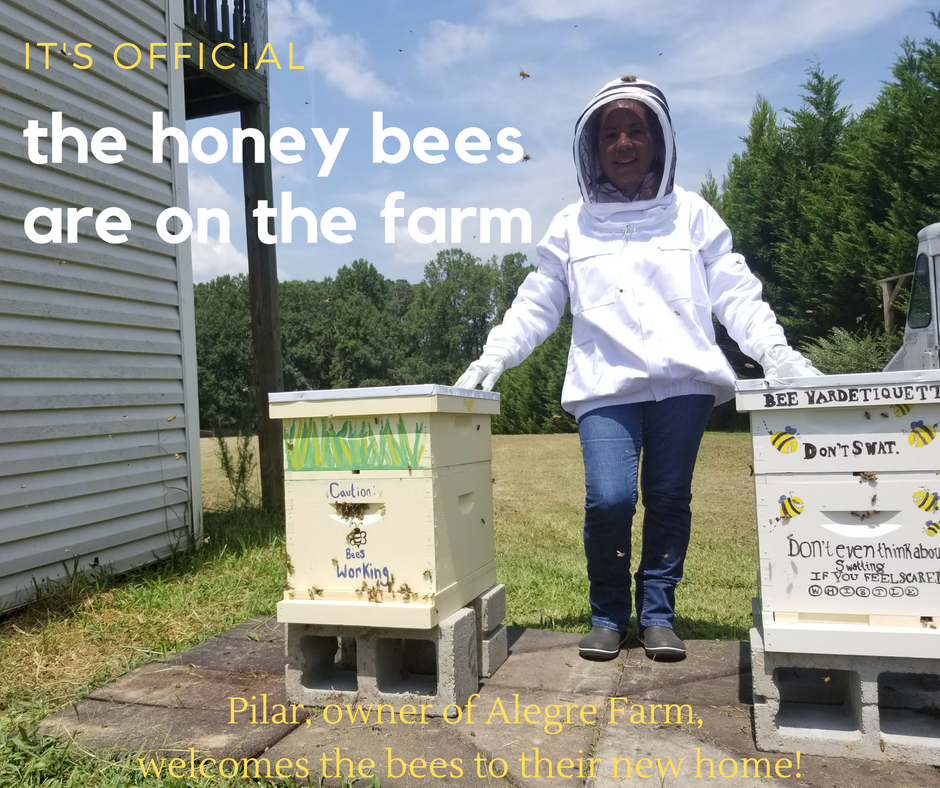Last Wednesday, we brought home our honey bees! It's so exciting to have them back on the farm buzzing around!

As we have been preparing our garden for fall, we noticed an increase in the number of honey bees on the flowers, so we know they are hard at work preparing for the winter ahead!

Watch our video as Pilar transfers the bees into their new hive here on Facebook!

 table div table+table+table+table+table+table+table div table{width:100%;padding:0}table div table+table+table+table+table+table+table div table img{width:96.23%;padding:0;float:none}table div table+table+table+table+table+table+table div table td{width:100%;padding:0 1.88% 18px}/* styles */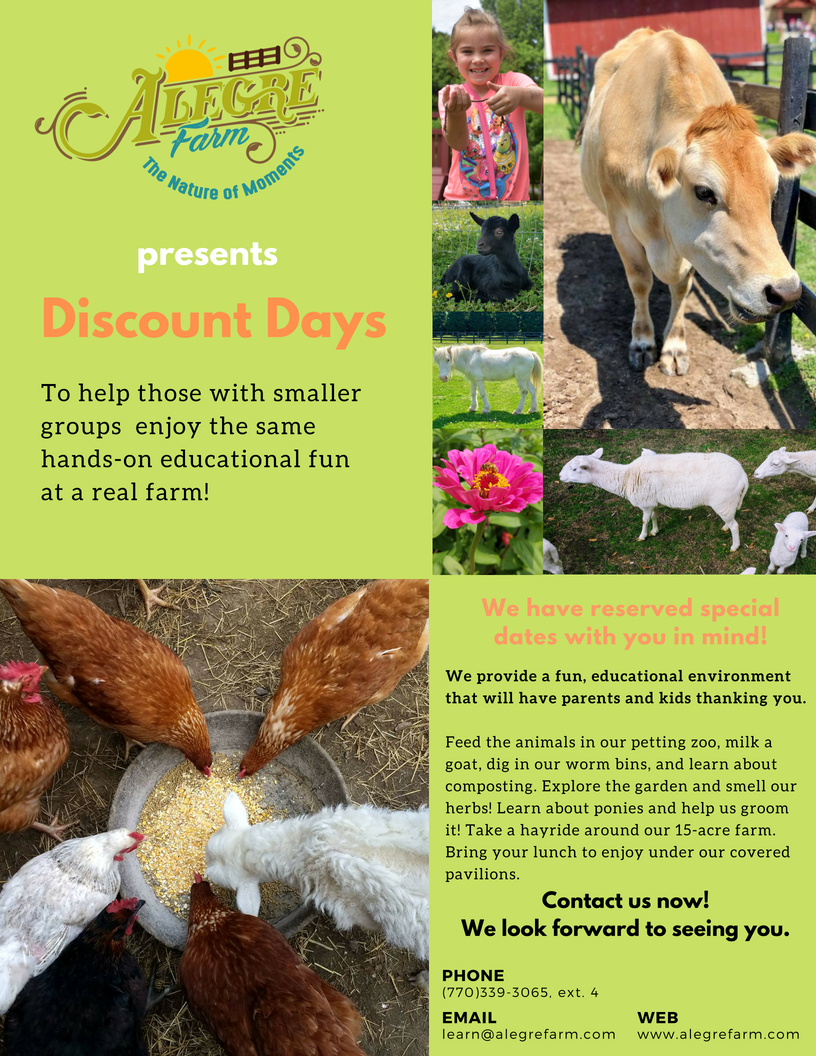# Discount Days at the Farm!

In consideration of those who may have smaller groups, Alegre Farm has decided to create specific dates which attendees can come at a discounted rate. As we finalize the schedule, we will let you in the coming days what days it will be held!

If you would like to be notified of these dates by e-mail, please contact learn@alegrefarm.com.

 table div table+table+table+table+table+table+table+table+table div table{width:100%;padding:0}table div table+table+table+table+table+table+table+table+table div table img{width:96.23%;padding:0;float:none}table div table+table+table+table+table+table+table+table+table div table td{width:100%;padding:0 1.88% 18px}/* styles */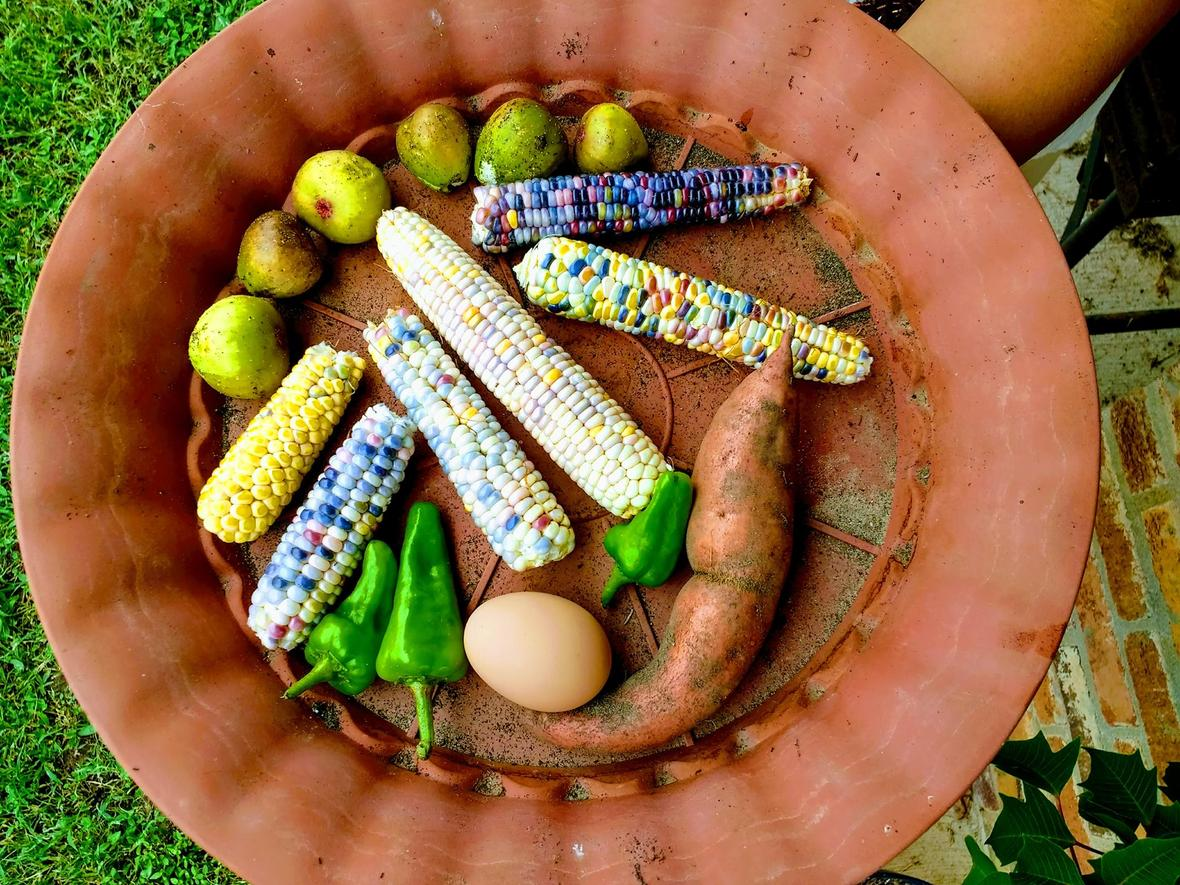# A Beautiful Harvest of Colorful Corn!

The Special Needs Group of Gwinnett have joined Alegre Farm to volunteer in our garden on Mondays.

This week they did a great job and helped us pick some delicious food. We all loved the corn with its array of delightful color!

 table div table+table+table+table+table+table+table+table+table+table+table div table{width:100%;padding:0}table div table+table+table+table+table+table+table+table+table+table+table div table img{width:96.23%;padding:0;float:none}table div table+table+table+table+table+table+table+table+table+table+table div table td{width:100%;padding:0 1.88% 18px}/* styles */## We're Still LookingWe are seeking teachers to guide students through interactive learning stations in our gardens and with our animals. Teaching and farm experience is helpful, but not required.

Please contact our Education Director, Angie Mallard, for more information. Email: learn@alegrefarm.com
Phone: (770) 339-3065, extension 4.

 table div table+table+table+table+table+table+table+table+table+table+table+table+table+table+table div table{width:100%;padding:0}table div table+table+table+table+table+table+table+table+table+table+table+table+table+table+table div table img{width:96.23%;padding:0;float:none}table div table+table+table+table+table+table+table+table+table+table+table+table+table+table+table div table td{width:100%;padding:0 1.88% 18px}/* styles */## Part I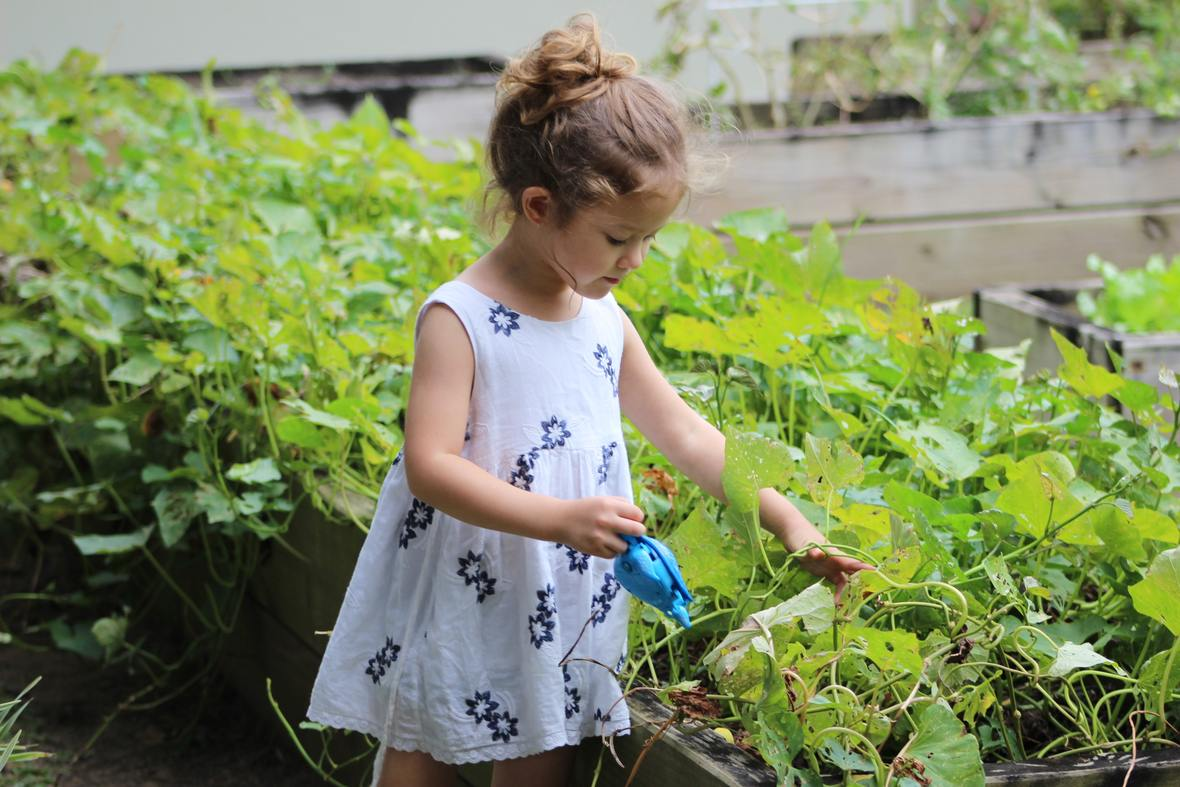## Grow Your Food Together!

Research has shown that a child is 5x's more likely to eat vegetables if they have grown it themselves.

So why not make it a family event that you can enjoy with your child to encourage them to eat more healthily? If you don't have a garden bed, you can start one in a container.

You can make a trip together and pick out the seeds. Then make it more fun with a pot you can paint to add your own flair.

This can also be an opportunity to connect with your child while instilling healthy habits!

Check out next week to find more ways to implement healthy eating at home!

 /* styles */ Click here to follow us on Facebook.
 table div table+table+table+table+table+table+table+table+table+table+table+table+table+table+table+table+table+table+table+table+table div table{width:100%;padding:0}table div table+table+table+table+table+table+table+table+table+table+table+table+table+table+table+table+table+table+table+table+table div table img{width:96.23%;padding:0;float:none}table div table+table+table+table+table+table+table+table+table+table+table+table+table+table+table+table+table+table+table+table+table div table td{width:100%;padding:0 1.88% 18px}/* styles */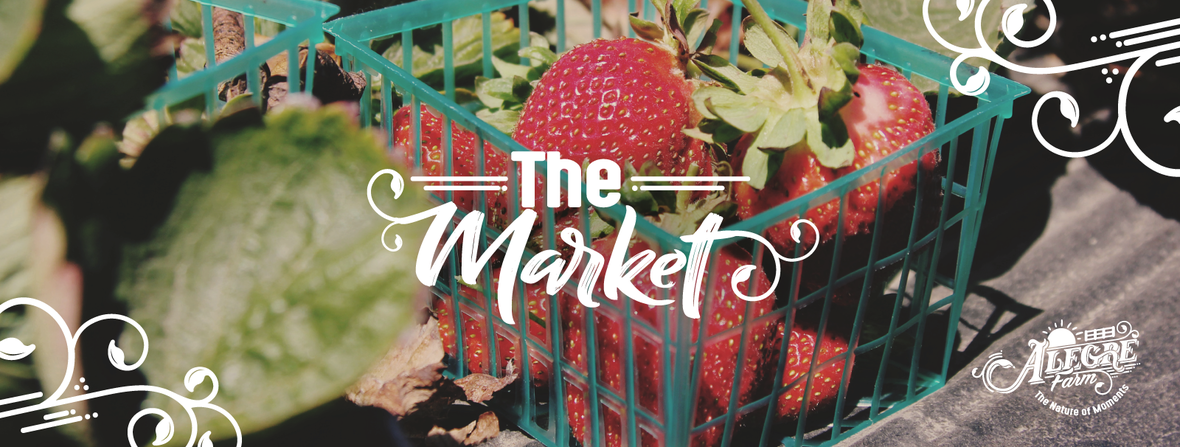## Support Your Local Farms and Businesses

We provide the community convenient access to nutrient dense, organically grown foods and healthy products. We are adding new items all the time so please check back weekly.

 table div table+table+table+table+table+table+table+table+table+table+table+table+table+table+table+table+table+table+table+table+table+table+table+table+table div table{width:100%;padding:0}table div table+table+table+table+table+table+table+table+table+table+table+table+table+table+table+table+table+table+table+table+table+table+table+table+table div table img{width:96.23%;padding:0;float:none}table div table+table+table+table+table+table+table+table+table+table+table+table+table+table+table+table+table+table+table+table+table+table+table+table+table div table td{width:100%;padding:0 1.88% 18px}/* styles *//* styles */ Please remember to order by 5pm Wednesdays for the same week pick up.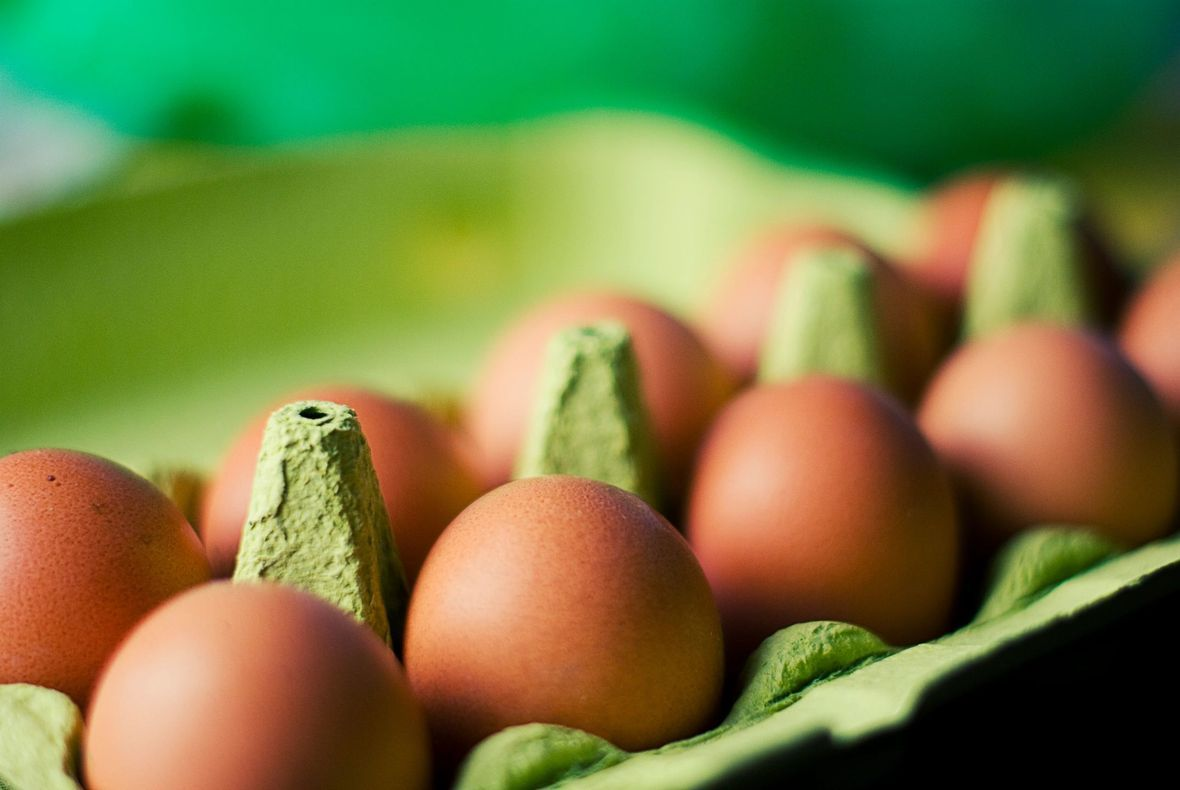Farm Fresh EggsFresh Raw Milk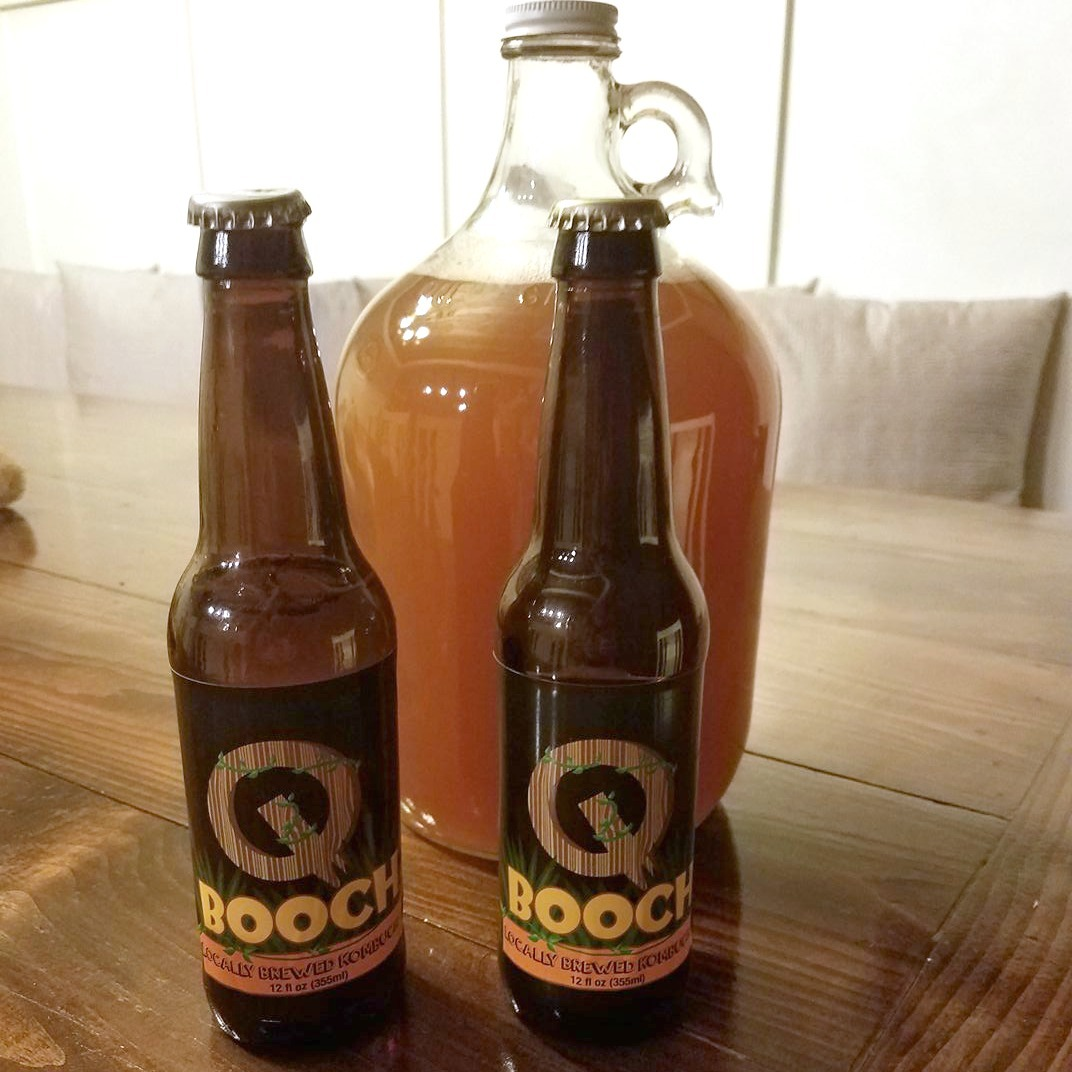Locally Brewed Kombucha
 /* styles */ If you have any questions about our market or ordering, please contact grow@alegrefarm.com
 table div table+table+table+table+table+table+table+table+table+table+table+table+table+table+table+table+table+table+table+table+table+table+table+table+table+table+table+table+table+table div table{width:100%;padding:0}table div table+table+table+table+table+table+table+table+table+table+table+table+table+table+table+table+table+table+table+table+table+table+table+table+table+table+table+table+table+table div table img{width:96.23%;padding:0;float:none}table div table+table+table+table+table+table+table+table+table+table+table+table+table+table+table+table+table+table+table+table+table+table+table+table+table+table+table+table+table+table div table td{width:100%;padding:0 1.88% 18px}/* styles */## Alegre Farm is an exceptional event space to hold your next gathering!

Now that team sports are in play, let us help you organize your end of season celebration!
We have options to provide the catering and entertainment. Let us know what you need!

Questions? Click here to fill out the form and we'll be in touch!

## We can host it!

We can give your child an amazing birthday party with pony rides and a bounce house to make them feel so special!
We hold family reunions, baby showers, corporate events, and weddings! We can please all crowds, young and old!

Contact events@alegrefarm.com to have your next event with us!

 table div table+table+table+table+table+table+table+table+table+table+table+table+table+table+table+table+table+table+table+table+table+table+table+table+table+table+table+table+table+table+table+table+table div table{width:100%;padding:0}table div table+table+table+table+table+table+table+table+table+table+table+table+table+table+table+table+table+table+table+table+table+table+table+table+table+table+table+table+table+table+table+table+table div table table{padding:0;float:left!important;width:34.716%!important}table div table+table+table+table+table+table+table+table+table+table+table+table+table+table+table+table+table+table+table+table+table+table+table+table+table+table+table+table+table+table+table+table+table div table table+table+table td,table div table+table+table+table+table+table+table+table+table+table+table+table+table+table+table+table+table+table+table+table+table+table+table+table+table+table+table+table+table+table+table+table+table div table td{padding-left:0;padding-right:0}table div table+table+table+table+table+table+table+table+table+table+table+table+table+table+table+table+table+table+table+table+table+table+table+table+table+table+table+table+table+table+table+table+table div table table td,table div table+table+table+table+table+table+table+table+table+table+table+table+table+table+table+table+table+table+table+table+table+table+table+table+table+table+table+table+table+table+table+table+table div table table+table td{padding-left:0;padding-right:20px}table div table+table+table+table+table+table+table+table+table+table+table+table+table+table+table+table+table+table+table+table+table+table+table+table+table+table+table+table+table+table+table+table+table div table table+table{float:left!important;width:34.528%!important}table div table+table+table+table+table+table+table+table+table+table+table+table+table+table+table+table+table+table+table+table+table+table+table+table+table+table+table+table+table+table+table+table+table div table table+table+table{float:left!important;width:30.756%!important}/* styles */
 table div table+table+table+table+table+table+table+table+table+table+table+table+table+table+table+table+table+table+table+table+table+table+table+table+table+table+table+table+table+table+table+table+table+table div table{width:100%;padding:0}table div table+table+table+table+table+table+table+table+table+table+table+table+table+table+table+table+table+table+table+table+table+table+table+table+table+table+table+table+table+table+table+table+table+table div table img{width:96.23%;padding:0;float:none}table div table+table+table+table+table+table+table+table+table+table+table+table+table+table+table+table+table+table+table+table+table+table+table+table+table+table+table+table+table+table+table+table+table+table div table td{width:100%;padding:0 1.88% 18px}/* styles *//* styles */ Kind regards, Your Team at Alegre Farm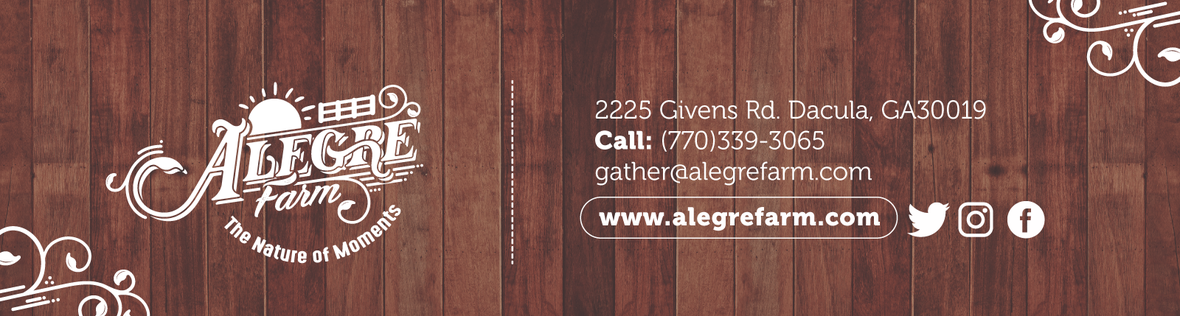Like   Tweet   Pin   +1   in